Ex 13.1

Chapter 13 Class 9 Surface Areas and Volumes (Term 2)
Serial order wise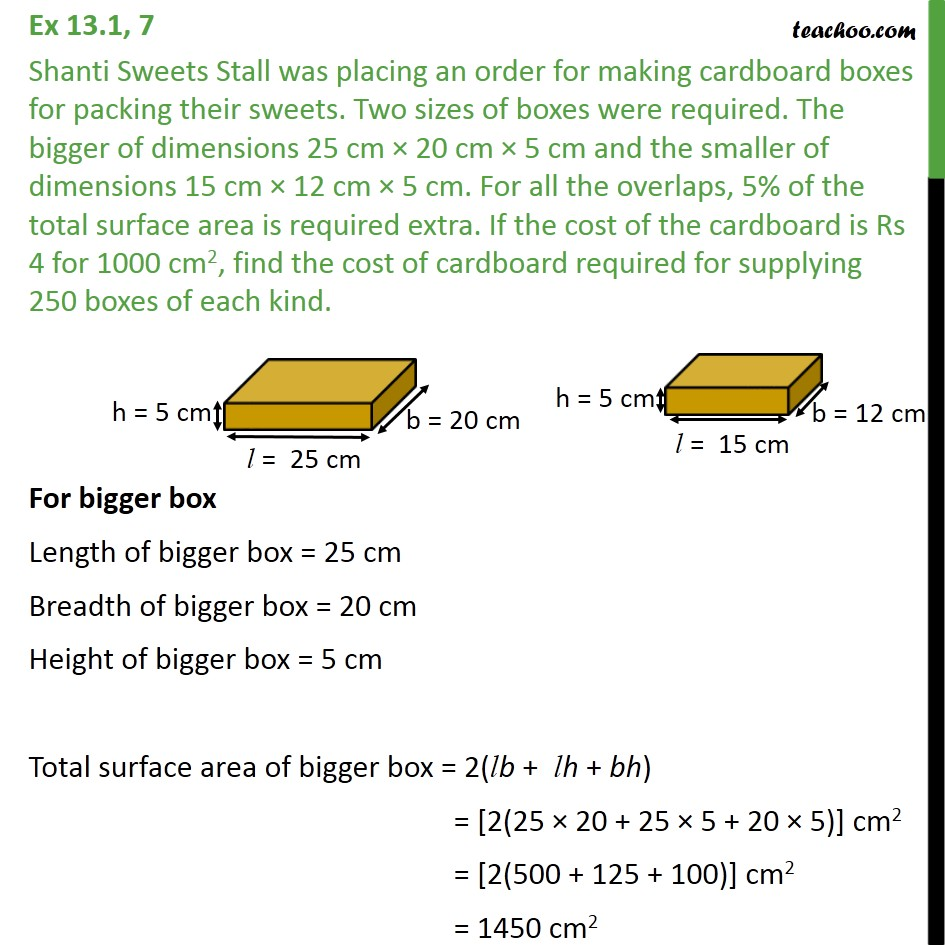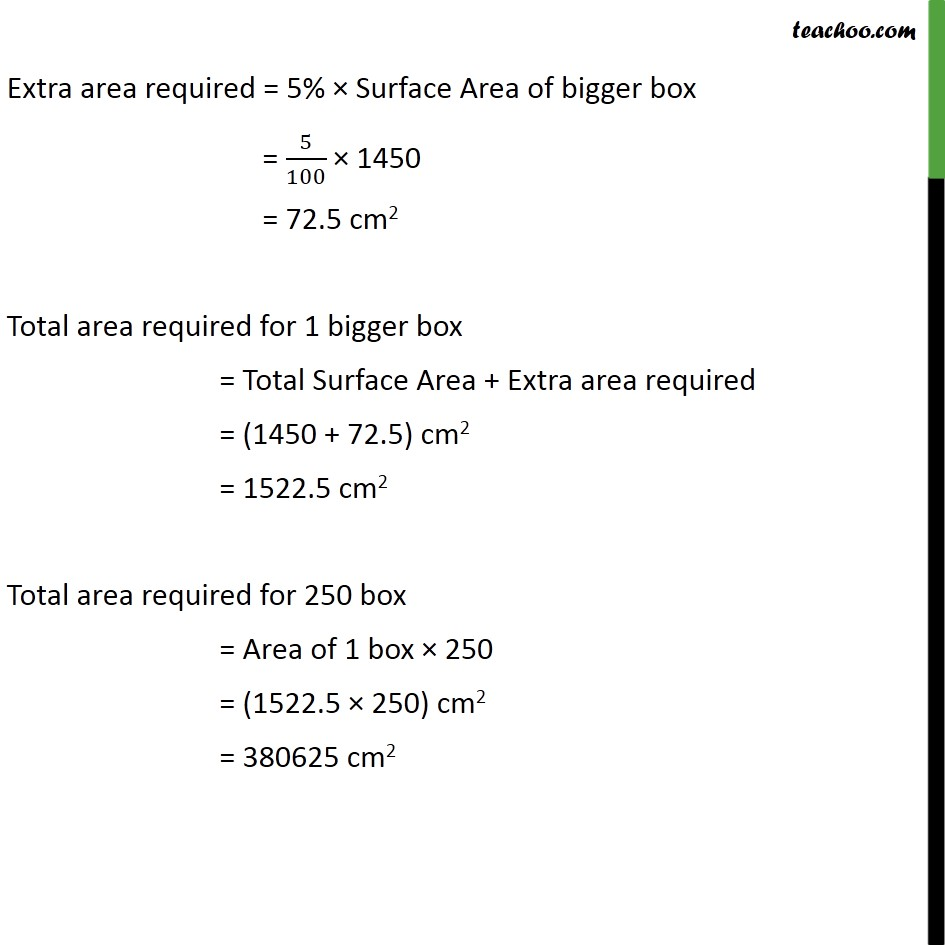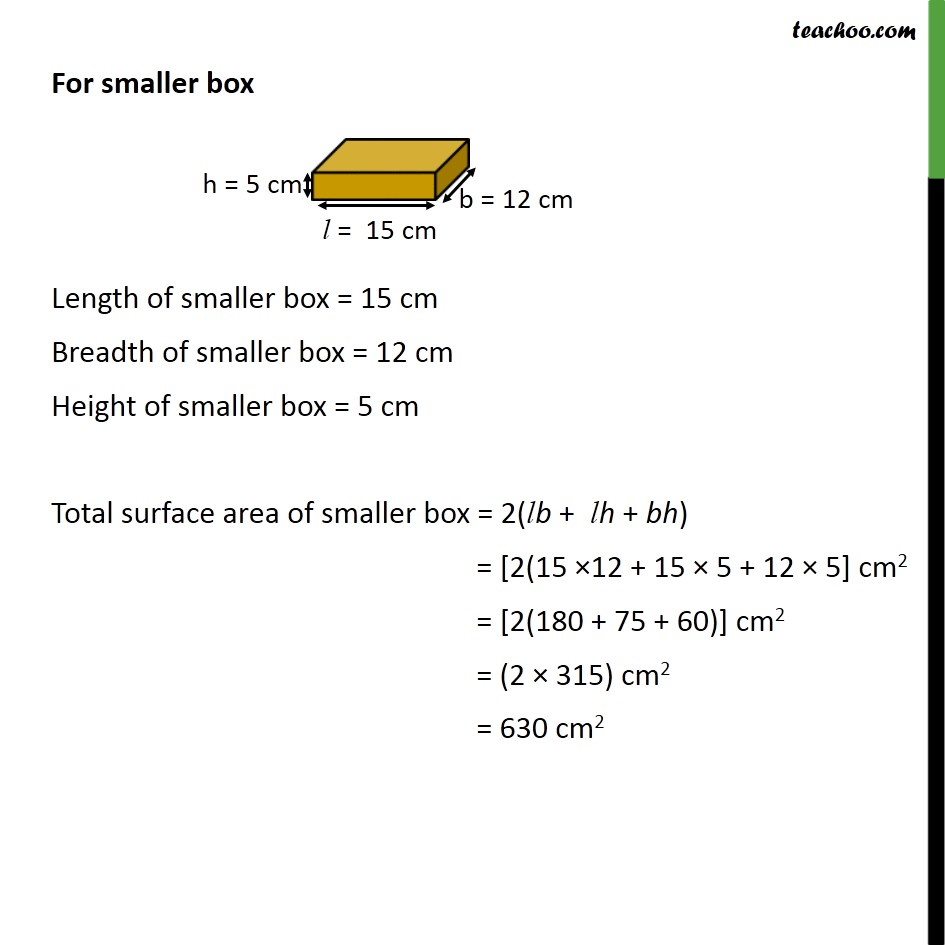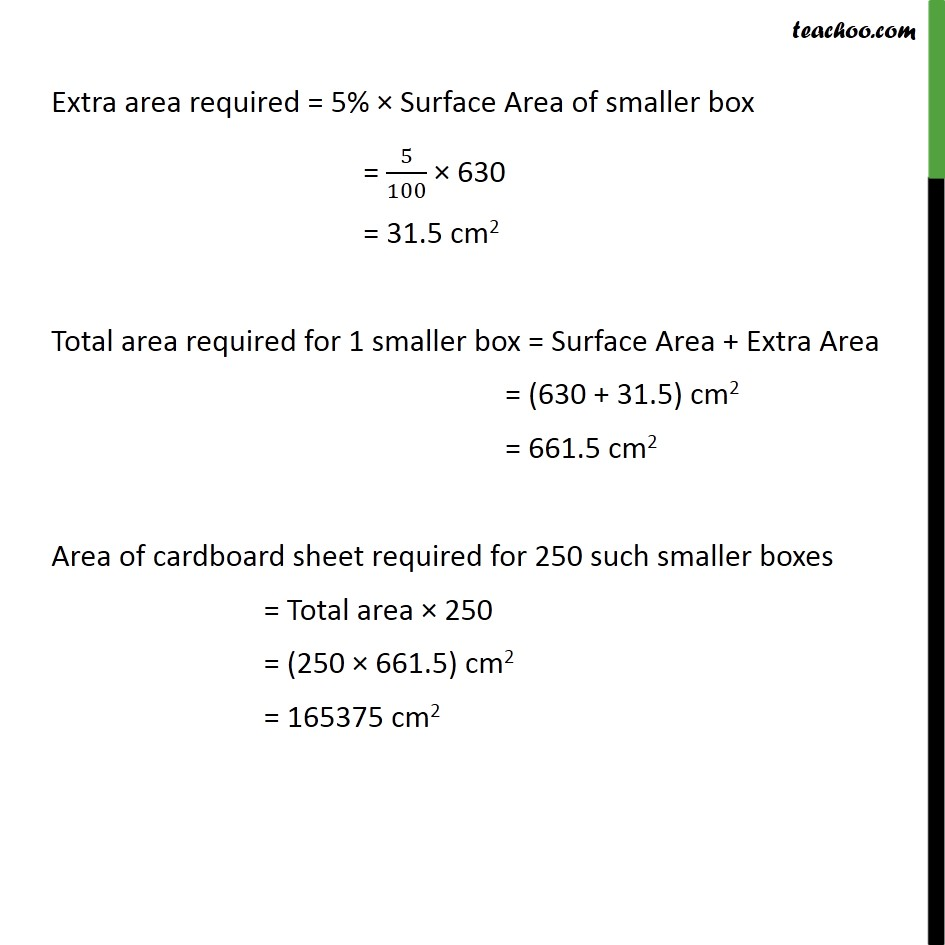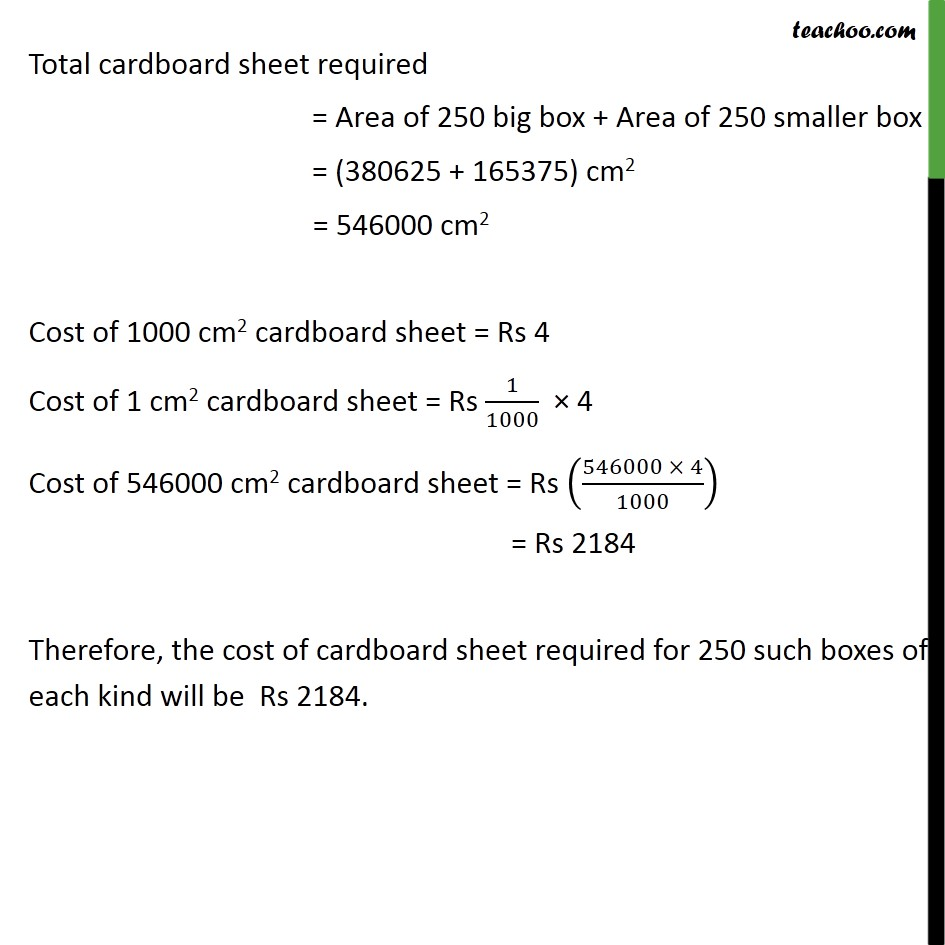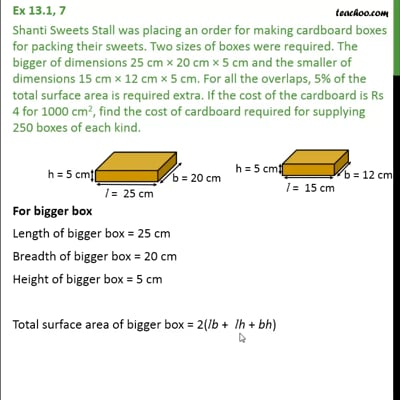This video is only available for Teachoo black users

### Transcript

Ex 13.1, 7 Shanti Sweets Stall was placing an order for making cardboard boxes for packing their sweets. Two sizes of boxes were required. The bigger of dimensions 25 cm × 20 cm × 5 cm and the smaller of dimensions 15 cm × 12 cm × 5 cm. For all the overlaps, 5% of the total surface area is required extra. If the cost of the cardboard is Rs 4 for 1000 cm2, find the cost of cardboard required for supplying 250 boxes of each kind. For bigger box Length of bigger box = 25 cm Breadth of bigger box = 20 cm Height of bigger box = 5 cm Total surface area of bigger box = 2(lb + lh + bh) = [2(25 × 20 + 25 × 5 + 20 × 5)] cm2 = [2(500 + 125 + 100)] cm2 = 1450 cm2 Extra area required = 5% × Surface Area of bigger box = 5/100 × 1450 = 72.5 cm2 Total area required for 1 bigger box = Total Surface Area + Extra area required = (1450 + 72.5) cm2 = 1522.5 cm2 Total area required for 250 box = Area of 1 box × 250 = (1522.5 × 250) cm2 = 380625 cm2 For smaller box Length of smaller box = 15 cm Breadth of smaller box = 12 cm Height of smaller box = 5 cm Total surface area of smaller box = 2(lb + lh + bh) = [2(15 ×12 + 15 × 5 + 12 × 5] cm2 = [2(180 + 75 + 60)] cm2 = (2 × 315) cm2 = 630 cm2 Extra area required = 5% × Surface Area of smaller box = 5/100 × 630 = 31.5 cm2 Total area required for 1 smaller box = Surface Area + Extra Area = (630 + 31.5) cm2 = 661.5 cm2 Area of cardboard sheet required for 250 such smaller boxes = Total area × 250 = (250 × 661.5) cm2 = 165375 cm2 Total cardboard sheet required = Area of 250 big box + Area of 250 smaller box = (380625 + 165375) cm2 = 546000 cm2 Cost of 1000 cm2 cardboard sheet = Rs 4 Cost of 1 cm2 cardboard sheet = Rs 1/1000 × 4 Cost of 546000 cm2 cardboard sheet = Rs ((546000 × 4)/1000) = Rs 2184 Therefore, the cost of cardboard sheet required for 250 such boxes of each kind will be Rs 2184.